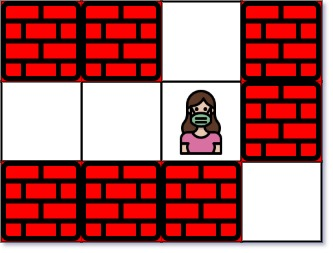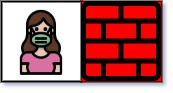# Nearest Exit from Entrance in Maze LeetCode Solution

Difficulty Level Medium

## Problem Statement

Nearest Exit from Entrance in Maze LeetCode Solution – We are given an m x n matrix “maze” (0-indexed) with empty cells represented as ‘.’ and walls as ‘+’. You are also given the entrance of the maze, where entrance = [entrance_row, entrance_col] denotes the row and column of the cell you are initially standing at.

In one step, we can move one cell updownleft, or right. We cannot step into a cell with a wall, and cannot step outside the maze. Our goal is to find the nearest exit from the entrance. An exit is defined as an empty cell that is at the border of the maze. The entrance does not count as an exit.

We are asked to return the number of steps in the shortest path from the entrance to the nearest exit, or -1 if no such path exists.

## Examples & Explanations

Example 1:```Input: maze = [["+","+",".","+"],[".",".",".","+"],["+","+","+","."]], entrance = [1,2]
Output: 1
Explanation: There are 3 exits in this maze at [1,0], [0,2], and [2,3].
Initially, you are at the entrance cell [1,2].
- You can reach [1,0] by moving 2 steps left.
- You can reach [0,2] by moving 1 step up.
It is impossible to reach [2,3] from the entrance.
Thus, the nearest exit is [0,2], which is 1 step away.```

Example 2:```Input: maze = [["+","+","+"],[".",".","."],["+","+","+"]], entrance = [1,0]
Output: 2
Explanation: There is 1 exit in this maze at [1,2].
[1,0] does not count as an exit since it is the entrance cell.
Initially, you are at the entrance cell [1,0].
- You can reach [1,2] by moving 2 steps right.
Thus, the nearest exit is [1,2], which is 2 steps away.```

Example 3:```Input: maze = [[".","+"]], entrance = [0,0]
Output: -1
Explanation: There are no exits in this maze.```

## Approach

Let’s consider we are somewhere in the maze and are not on the outermost layer of the maze. The fastest way to exit the matrix is to travel all the possible cells from our initial position and take the shortest exit. We have to take care of an edge case where our initial position is at an outermost cell in the maze. While exiting, we need to check if entrance == exit, if yes, we can’t exit from the maze otherwise it’s the answer.

We will use BFS to traverse all the possible cells from the initial position. Traverse all the elements present in the queue at a time because moving to any of these cells will take equal distance. We will run a while loop for sz times where sz = size of the queue. Then we will check all possible cells we can move to. All possible directions are defined in another variable dir. If the move is allowed, we will move to that cell and store it in the queue.

The move is allowed iff:

1. coordinates x and y ≥ 0
2. x < m & y < n
3. cell[x][y] == ‘.’

## Code

### C++ code for Nearest Exit from Entrance in Maze

```#define iPair pair<int,int>
#define deb(x) cout<<#x<<" = "<<x
class Solution {
int dir = {{0,-1}, {-1,0}, {0,1}, {1,0}};
public:
int nearestExit(vector<vector<char>>& maze, vector<int>& entrance) {
int m = maze.size(), n = maze.size();
int moves=1;
queue<iPair> q;
q.push({entrance, entrance});

while(!q.empty()) {
int sz = q.size();
while(sz--) {
auto curr=q.front();
q.pop();

for(auto d: dir) {

int x = curr.first + d, y = curr.second + d;

if(x >= 0 && x < m && y >= 0 && y < n && maze[x][y] != '+'){

if(x == 0 || x == m-1 || y==0 || y==n-1) {
if(!(x==entrance && y == entrance))
return moves;
}

q.push({x, y});
maze[x][y] = '+';
}

}
}
moves++;
// deb(moves);
}

return -1;
}
};```

### Java code for Nearest Exit from Entrance in Maze

```class Solution {
int[][] dirs = {{0,1},{0,-1},{1,0},{-1,0}};
public int nearestExit(char[][] maze, int[] entrance) {
int rows = maze.length, cols = maze.length;
boolean[][] visited = new boolean[rows][cols];
int[] curr;

int x, y, moves = 0;

q.offer(entrance);
visited[entrance][entrance] = true;

while (!q.isEmpty()) {
int sz = q.size();
moves++;

for (int i=0; i<sz; i++) {
curr = q.poll();

for (int[] dir: dirs) {
x = dir+curr;
y = dir+curr;

if (x<0||x>=rows||y<0||y>=cols) continue;

if (visited[x][y] || maze[x][y] == '+') continue;

if (x==0||x==rows-1||y==0||y==cols-1) return moves;

q.offer(new int[]{x, y});
visited[x][y] = true;
}
}
}

return -1;
}
}```

## Complexity Analysis for Nearest Exit from Entrance in Maze LeetCode Solution

• Time complexity: O(m*n)
• In the worst case, we need to traverse from the first cell to the last cell
• The size of the matrix is m x n, hence total time is O(m*n)
• Space complexity: O(m*n)
• The visited 2D matrix takes m x n space
Translate »